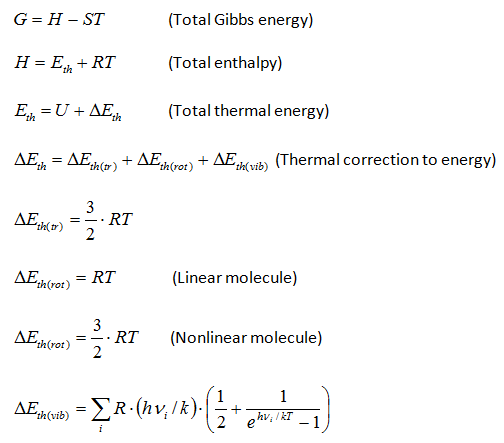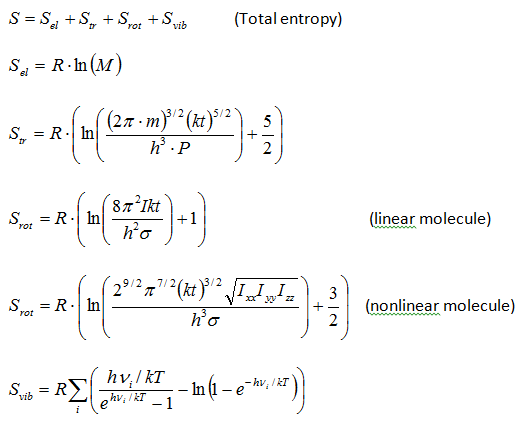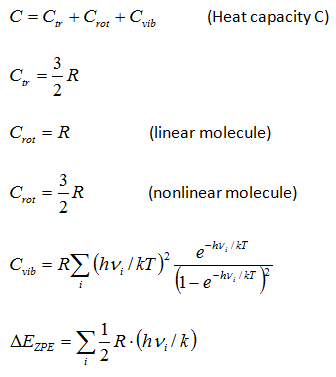## Thermodynamic functions calculator

Chemcraft can compute the entropy and the Gibbs energy of a molecule by its molecular parameters, including the geometry (moments of inertia) and vibrational frequencies. This computation is made in "rigid rotor - harmonic oscillator" approximation (RRHO). The main aim of this utility is the possibility to cut low frequencies, which produce big errors because of ahharmonicity. You can specify a threshold value, e.g. 100 cm**-1, and the values of the frequencies below this threshold will be increased to this threshold value. The formulas for the computation were taken from the following refs.:

Donald A. McQuarrie, John D. Simon. Molecular Thermodynamics. University Science Books, 1999.

Thermochemistry in Gaussian. Joseph W. Ochterski, Ph.D. June 2, 2000. https://gaussian.com/thermo/

ASE thermochemistry computation. https://wiki.fysik.dtu.dk/ase/ase/thermochemistry/thermochemistry.html

Here are the formulas implemented in Chemcraft:Ā
Other functions:ĀYou can download the Delphi source code which computes the thermodynamic functions in Chemcraft here: chemdata_u_b646.zip.

Troubleshooting
Currently this utility in Chemcraft does not always determine correctly the point
groups of highly-symmetrical molecules (point groups T, Td, Th, O, Oh, I, Ih), so the Srot values can be computed improperly correctly for such molecules. You should check the point group in the printout. Before applying the frequencies threshold, we recommend to compare the S, C, G values printed by Chemcraft (without using the frequencies threshold) with the values printed by your QC package. We have performed such a comparison for a number of Gaussian and Orca output files. For Gaussian, the results are almost equal, except some molecules with point groups T, Td, Th, O, Oh, I, Ih. For Orca, the values of most thermodynamic functions are almost equal, but the Svib values are slightly different; this is caused by the fact that the version of Orca we used (5.0.4) uses the Svib computation algorithm other than the standard RRHO (in Orca, the QRRHO approach is implemented).

Experimental verification of the Gibbs energies computation

We have performed a computation of pKa values (pKa=Ga/2.303RT) of some carboxylic acids and phenols, which can be compared with experimental ones. The set of compounds studies included 21 carbon and benzoic acids, and 14 phenols. The pKa values do not directly match the experimental ones from literature (this is common), but the linear fits can be obtained. The molecules were computed via Orca 5.0.3 package with method PCM B3LYP/6-31++G(D,P) (with explicit water molecules). The mean absolute deviations (mean differences between the experimental pKa values and the values calculated by fit parameters) are in the range of 0.12 - 0.6 pKa units. For RRHO computations of Gibbs energies (implemented in Chemcraft) of carboxylic acids, the MADs obtained are 0.21 pKa units without the frequencies threshold, 0.15 with the threshold 40 cm**-1, 0.14 with 100 cm**-1 threshold, 0.12 with 300 cm**-1 threshold, and 0.13 with 800 cm**-1 threshold. For RRHO computations of phenols, the MADs are 0.60 pKa units without the threshold, 0.41 with 40 cm**-1, 0.35 with 100 cm**-1, 0.28 with 300 cm**-1 and 0.29 with 800 cm**-1 threshold. For QRRHO computations (implemented in Orca) of carbon acids, the MADs obtained are 0.17 pKa units without the threshold and 0.13 with 100 cm**-1 threshold; QRRHO computations of phenols produced the MADs 0.44 without the threshold and 0.33 with 100 cm**-1 threshold. So, our study confirms that applying the threshold of 100-300 cm**-1 is a good approach which improves the agreement of computed Gibbs energies with the experiment. We plan to publish these results.

BACK